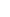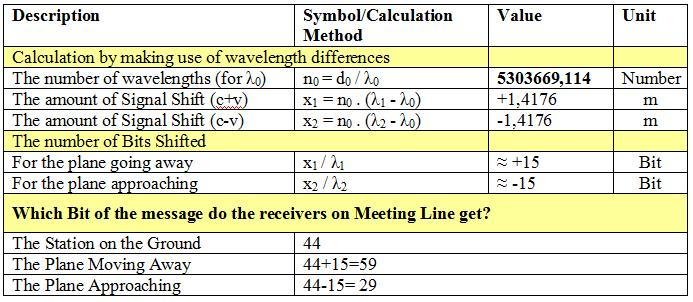16. THE FORTY-FORTH BIT (The 44th BIT)Id like to conclude the topic of Byte Shift with an example using real values. The results we will get here are important because the things we will see here will make it a lot easier to understand the topics in the queue. On the other hand, the use of real values while covering the topic will precisely show us how the event of Byte Shift occurs.The set-up of the event closely resembles the previous ones that we have seen. While the transmitter is sending the message Hello World, the planes are moving towards Meeting Line (see the figure above).We will deal with a special situation where the distances of all three signal receivers to the transmitter are equal when the planes are at Meeting Line (see the figure below).Lets assume that, at this moment, the station on the mountain receives the 44th bit of the message. In this case, which Bit of the message that is coming to them will the planes be receiving? This is our topic. We will do the necessary calculations to answer this question.The 44th Bit falls in the middle of the message that consists of 88 Bits.  Lets see the situation in a figure. We are going to find the locations of the 44th bit shown with a question mark relative to the meeting line in the figure below for the message going to the planes.We use the following table for base data and calculations:Information on message groups:We can use two methods to calculate where the 44th Bit is for the planes.  Calculation method by using the number of wavelengths  Lets assume that the message Hello World is a message that starts as Hello World. Welcome to  .. and has infinite length. As each wavelength belonging to the message carries data of 1 Bit, we can do calculations by making use of the number of wavelengths number between the receiver and the transmitter.  Calculation method by making use of signal speeds  We can do calculations by using the travel time of the signal that goes from the transmitter to the station on the mountain and the speeds of signal groups.  We see the calculations done by each method in the table below.Lets try to complete the previous figure with the information we obtained.In this way, we see how Byte Shift takes place. Although the receivers are at the same distance from the transmitter, the station on the mountain, the plane going away and the plane approaching receive a different part of the message at that moment. While the transmitter on the mountain gets the character   (Space), the plane going away gets O character and the plane approaching gets L character.Im going to end this part about Byte Shift with a reminder. It is crucial to remember that (c+v) (c-v) mathematics takes place between two objects. At the moment the signal emitted reaches its target, its journey is over. The previous figures were illustrated to cover the topic and do the calculations and, in those illustrations, it seems as if the signals keep traveling after they reach their targets. Of course, this doesnt happen. The illustration reflecting the reality is below. The journey is over for the signal parts that reach the receiver.We can directly find which Bit of the message the planes receive at Meeting Line with a third calculation method based on wavelength differences.